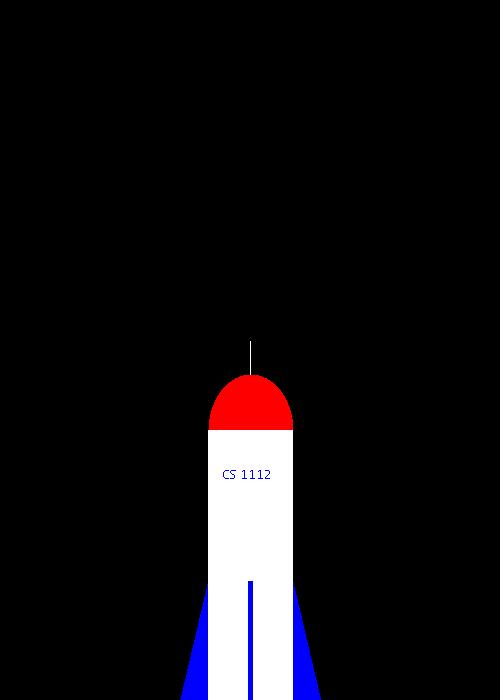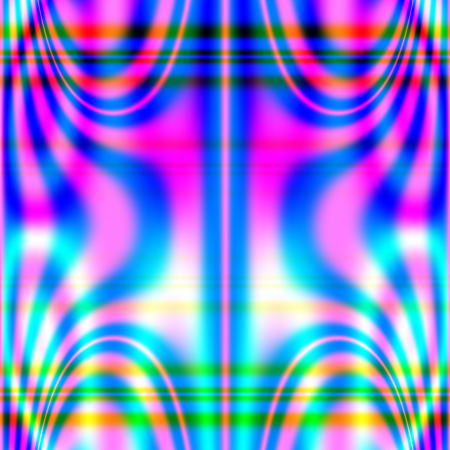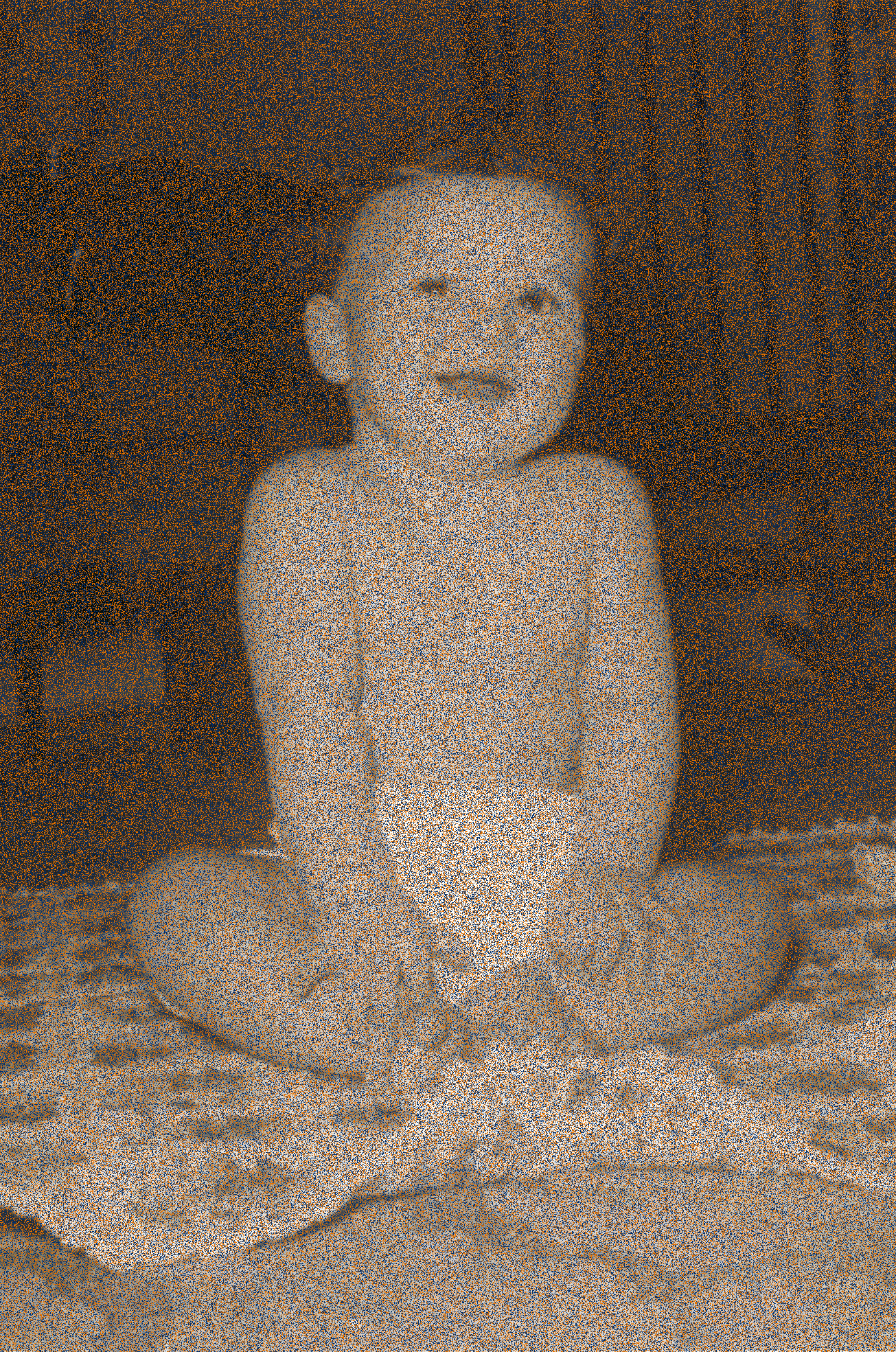Class 28 — Friday November 2

TBD

Agenda

• Start image manipulation

Discussion

• Pillow is a library for manipulating images in Python. Unlike the math library and others we have used, Pillow is not part of the standard Python install; you have to add it yourself.
• To install Pillow on your laptop go here.
• For now the parts of Pillow of interest to us are:
• Image: Pillow representation of an image.
• The Image has one particular function of current interest to us:
• `Image.new( m, size, color='black' )` returns a mode m new image, whose dimensions are `size`. The background of the image color. Parameter color is optional with a default value of black. For us,
• `m` will always be the string `'RGB'`;
• `size` will always be an ordered pair of a list of the form `[ w, h ]`, where `w` is the width of the image and `h` is the height of the image.

Homework 22

• Define a function `picture()` that returns an interesting image. The function takes no parameters.
• Progam checkout.py will invoke your `picture()` function and save the result as `myart.jpg1
• Submit `artistry.py` for credit on this assignment.
• You may optionally also submit the picture file `myart.jpg`. If you submit the picture, it will be eligible for a prize — if you do submit a picture, you are willing to let other people see it (e.g., it can be posted on UVA web sites). Submitting the picture is not part of your grade, but you are encouraged to submit.

Pillow epistle

• The primary package for support graphics and image manipulation is Pillow. Pillow is not part of the standard Python install; you have to add it yourself. To install Pillow on your laptop go here. The parts of Pillow of interest to us are:
• Image : Pillow representation of an image.
• To gain access to Pillow functionality the module `PIL` needs to be imported. The typical way to gain access to `Image` and `ImageDraw` is statement

from PIL import Image, ImageDraw

Image

• The Image function that we care about for now is its `new()` function — through it we can construct a new image.
• Function invocation `Image.new( m, size, color='Black' )` returns a mode `m` new image, whose dimensions are `size`; where the background of the image is filled-in with `color`. Parameter `color` is optional with a default value of black. The units of measure for the size are in pixels. For us, `m` will always be the string `'RGB'`; this mode uses differents levels of red, green, and blue to produce other colors.• Parameter `size` will always be an ordered pair of the form `( w, h )`, where `w` is the width of the image and `h` is the height of the image.
• The following code segment defines a new image object called `im`. The image is 550 pixels wide and 450 pixels high and its background color is midnight blue.

im_width = 480

im_height = 400

dimensions = [ im_width, im_height ]

im = Image.new( 'RGB', dimensions, color='Black' )

• The color names that Pillow knows about are the standard web colors. A list of them is available.
• An object of type `Image` has a method function `show()` that will create a pop-up window displaying its image.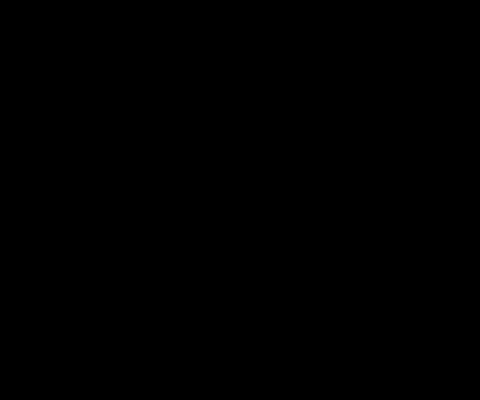• To see our new image do

im.show()

• To save a copy of an image use its method function `save()`.

im.save( 'canvas.jpg' )

Function `save()` expects a string parameter giving the name for the picture file. The file will be located in the same folder as the program that did the saving.

ImageDraw

• Given an `Image` object, you can access its drawing surface through function `ImageDraw.Draw()`.
• Suppose `im` is an image. My preference is to call the drawing surface `canvas`. The following statement initializes `canvas` to be the drawing surface for image `im`.

canvas = ImageDraw.Draw( im )

• The origin `( 0, 0 )` for a Pillow drawing is the upper-left-hand corner of the image.
• Most drawing commands in Pillow require an ordered-pair argument that gives the location and size of the shape. The first element of the pair is the location of the upper-left-hand corner of the shape; the second element is the lower-right-corner of the shape. The usual Pythonic name for the ordered-pair is `xy`.
• One such command is `ImageDraw` function `rectangle()`.
• The following code block indicates the shape is to be situated at `( x, y )` and extend to `( x+w, y+h )`.

x = 40

y = 80

w = 50

h = 75

xy = [ ( x, y ), ( x + w, y + h ) ]

canvas.rectangle( xy, outline='Cornsilk' )

Further, the shape is to be the outline of a rectangle with the outline colored green. Parameter `outline` is optional, if specified its value is to be the color of the perimeter.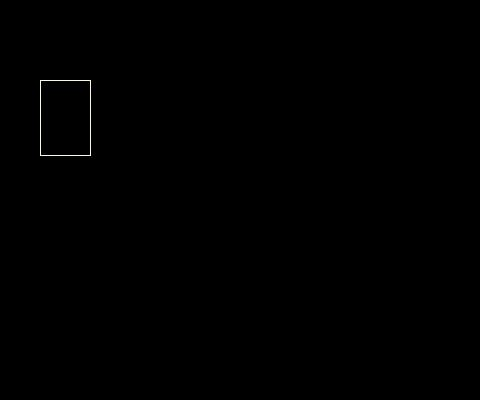• Another optional parameter is `fill`, if specified its value is to be the background color of the rectangle. For example,

x = 100

y = 20

w = 100

h = 25

xy = [ (x, y), (x + w, y + h) ]

canvas.rectangle( xy, fill='Lavender', outline='Orchid' )

Adds a filled-in rectangle to our drawing.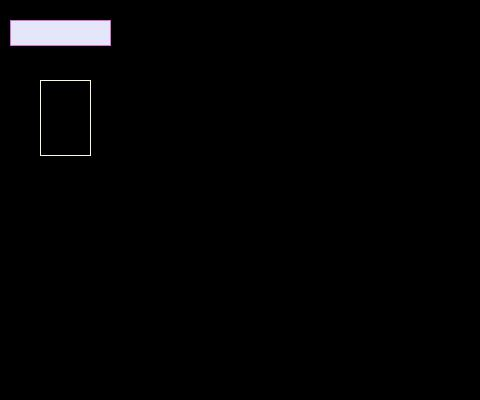• The function to draw an ellipse (oval) is `ImageDraw.ellipse()`. When drawing ellipses, the code specifies the upper-left-hand and lower-right-hand corners of the box that circumscribes the ellipse. * `fill` and `outline` parameters are optional parameters for `ImageDraw.oval()`
• The following code segment adds two ellipses to our drawing.

x = 120

y = 65

w = 150

h = 90

xy = [ (x, y), (x + w, y + h) ]

canvas.ellipse( xy, outline='Magenta' )

x = 300

y = 25

w = 50

h = 75

xy = [ (x, y), (x + w, y + h) ]

canvas.ellipse( xy, fill='DeepPink', outline='GhostWhite' )

Both ellipses have their perimeter drawn; and one also has its background coloorange red.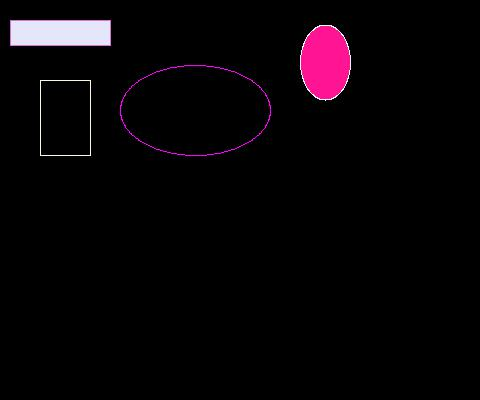• Pillow also provides the means to draw text through function `ImageDraw.text()`. The following code segment uses the function

coord = ( 25, 200 )

s = 'We are the best'

canvas.text( coord, s, fill='Moccasin' )

to add the string `'We are the best'` to our drawing. The first parameter to the function is the location to start the text; the second parameter is the desired text.• Another capability of `ImageDraw` is to draw a line through function `ImageDraw.line()`. The function requires an `xy` parameter and accepts optional parameters `fill` and `outline`. The following code segment

p0 = ( 25, 215 )

p1 = ( 115, 215 )

xy = [ p0, p1 ]

canvas.line( xy, s, fill='Moccasin' )

adds a horizontal line below our text.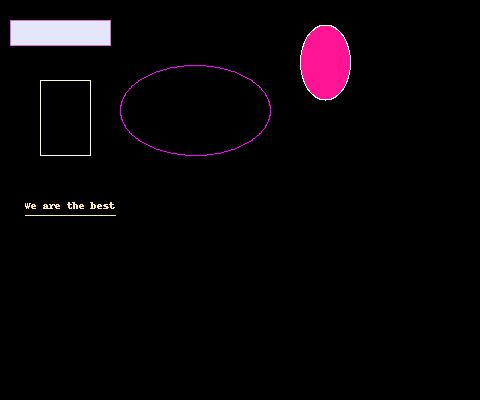• For drawing polygonal shapes `ImageDraw` has function `polygon()`. The function has one required parameter a list of locations `seq`. Optional parameters `fill` and `outline` are also available.
• The following code segment draws two polygons onto the canvas. The first polygon has its perimeter drawn; the second has its interior filled.

p0 = (350, 120)

p1 = (400, 140)

p2 = (425, 200)

p3 = (425, 260)

p4 = (375, 245)

p5 = (325, 190)

seq = [ p0, p1, p2, p3, p4, p5 ]

canvas.polygon( seq, outline='Peru' )

p0 = (175, 80)

p1 = (240, 110)

p2 = (190, 130)

p3 = (150, 120)

seq = [ p0, p1, p2, p3 ]

canvas.polygon( seq, fill='RebeccaPurple' )

• Afterwards the canvas now looks like• For drawing arcs of an ellipse use `ImageDraw` function `arc()`. The function has three required parameters `xy`, `a1`, and `a2`. Optional parameter `fill` can be used to specify the color of the arc. The function draws an arc within bounding box `xy` on the `canvas`. The arc outline has starting angle `a1` and ending angle `a2`.
• The following code segment adds a colored arc to the canvas.

p0 = ( 140, 220 )

p1 = ( 340, 380 )

xy = [ p0, p1 ]

a1 = 0

a2 = 90

canvas.arc( xy, a1, a2, fill='RoyalBlue' )

Note, a 0° angle points east; a 90° angle points south; a 180° angle points west; a 270° angle points north.

• Afterwards the canvas now looks like• The `ImageDraw` function `chord()` supports the drawing of a chord for an ellipse. A cord connects to points on an ellipse with a straight line. Like `arc()`, the function has three required parameters `xy`, `a1`, and `a2`. Optional parameter `fill` can be used to specify the background of the chord; and optional parameter `outline` to color its perimeter.
• The following code segment adds a chord to the canvas.

p0 = ( 140, 220 )

p1 = ( 340, 380 )

xy = [ p0, p1 ]

a1 = 150

a2 = 210

canvas.chord( xy, a1, a2, fill='MediumTurquoise' )

• Afterwards, the canvas looks like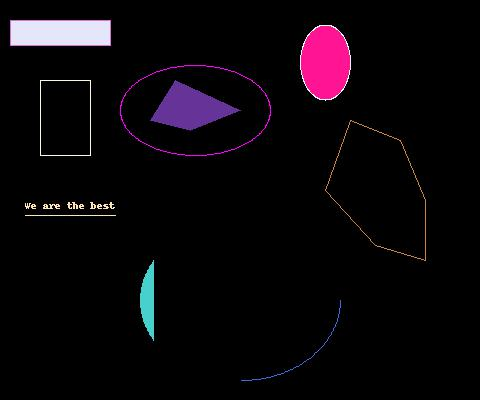• The `ImageDraw` function `pieslice()` supports the drawing of a pie slice for an ellipse. Like `arc()` and `chord()`, the function has three required parameters `xy`, `a1`, and `a2`. Optional parameter `fill` can be used to specify the background of the pie slice; and optional parameter `outline` to color its perimeter.
• The following code segment adds a pie slice to the canvas.

p0 = ( 140, 220 )

p1 = ( 340, 380 )

xy = [ p0, p1 ]

a1 = 225

a2 = 315

canvas.pieslice( xy, a1, a2, fill='OrangeRed' )

• Afterwards, the canvas looks like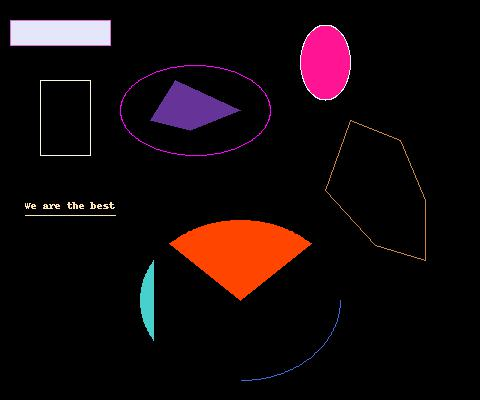To do

• Look over artifacts
• Do over homework
• Review Pillow drawing module
• Checkout past semester artistry highlights

Imagery